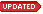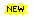# Free Math Practice Pages

 Resources Math for Kindergarten and Grade 1, such as A + B, A - B Math for Grade 2, 3, 4, such as A * B - C, A + B * C, with option of decimalMath for Grade 2, 3, division Math for Grade 3, 4, such as (A + B) * C, A * ( B - C) Math for Grade 4, 5, such as (A + B) * (A - B) = A*A - B*B, A*C +B*C = (A + B ) *C, A*C - B*C = (A-B) * C Math for Grade 3,4,5, fraction addition/subtraction with option of decimalMath for Grade 4,5, decimal addition/subtraction, multiplication Math for Grade 4, 5, 1-variable Algebra, More 1-variable Algebra Math for Grade 5, 6, 2-variable Algebra Math for Grade 4,5, division with remaining Math for Grade 4,5, Calculate GCD & LCM with option of algebra variablesMath for Grade 3,4,5, pattern, added a(2)=a(1)+a(0)Math for Grade 3,4,5, fraction sorting Math for Grade 5, 6, negative numbers, absolute values, exponentsMath for Grade 5, 6, absolute value equationMath for Grade 5, 6, inequality algebraMath for Grade 5, 6, equation factoringSuggested books for Math Competition Suggested books for SAT Preparation More coming soon... How to use those pages One refresh will create new set of exercises. Every exercise is fresh and random and is created on the fly. The answer for each exercise is hidden until you click the 'Flip Answers' on the top.To set different difficulty of the exercises, you can set your favorable mininum and maxinum values. Some answers have "Graph" linkswhich point to the powerful WolframeAlpha site. The equation of the exercise will be passed in as the parameter in the link. User are able to view the answer and graph of the equation.   Motivation to create those pages Save me tons of time and energy to search or prepare math practice exercises/worksheets for my kids Answer is always provided and saves me time to solve and verify. I am adding more from time to time in order to keep pace with my kids' acedemic needs. Your feedback will be welcomed and helpful. Last modified: 12/11/2011 Any questions or comments please contact us